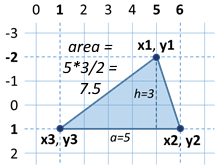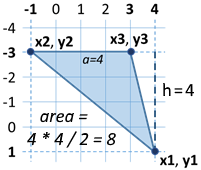Problem: Triangle Area

The first sample exam problem is about calculating the area of given triangle, specified by its coordinates (where the triangle stays horizontally).

Video: Triangle Area

Watch the video lesson about solving the triangle area problem: https://youtu.be/m2O8_rcNHtA.

Problem Description

 Triangle in the plane is defined by the coordinates of its three vertices. First the vertex (x1, y1) is set. Then the other two vertices are set: (x2, y2) and (x3, y3), which lie on a common horizontal line (i.e. they have the same Y coordinates). Write a program that calculates the area of the triangle by the coordinates of its three vertices.Input

The console provides 6 integers (one per line): x1, y1, x2, y2, x3, y3.

• All input numbers are in range [-1000 … 1000].
• It is guaranteed that y2 = y3.

Output

Print on the console the area of the triangle.

Sample Input and Output

5
-2
6
1
1
1
7.5The side of the triangle a = 6 - 1 = 5
The height of the triangle h = 1 - (-2) = 3
Triangle area S = a * h / 2 = 5 * 3 / 2 = 7.5
4
1
-1
-3
3
-3
8The side of the triangle a = 3 - (-1) = 4
The height of the triangle h = 1 - (-3) = 4
Triangle area S = a * h / 2 = 4 * 4 / 2 = 8

It is important in such types of tasks where some coordinates are given to pay attention to the order in which they are submitted, and to properly understand which of the coordinates we will use and how. In this case, the input is in order x1, y1, x2, y2, x3, y3. If we do not follow this sequence, the solution becomes wrong. First, we write the code that reads the input data:Calculate Triangle Side and Height

We have to calculate the side and the height of the triangle. From the pictures, as well as the condition y2 = y3, we notice that the one side is always parallel to the horizontal axis. This means that its length is equal to the length of the segment between its coordinates x2 and x3, which is equal to the difference between the larger and the smaller coordinates. Similarly, we can calculate the height. It will always be equal to the difference between y1 and y2 (or y3, as they are equal). Since we do not know if x2 is greater than x3, or y1 will be below or above the triangle side, we will use the absolute values of the difference to always get positive numbers, because one segment cannot have a negative length.Calculate and Print Triangle Area

we will calculate it using our familiar formula for finding an area of a triangle. An important thing to keep in mind is that although we get only integers at an input, the area will not always be an integer. That's why we use a variable of double type for the area. We have to convert the right side of the equation, because if we give whole numbers as equation parameters, our result will also be an integer.The only thing left is to print the area on the console.